## JAC Class 9 Maths Notes Chapter 1 संख्या पद्धति

Students should go through these JAC Class 9 Maths Notes Chapter 1 संख्या पद्धति will seemingly help to get a clear insight into all the important concepts.

### JAC Board Class 9 Maths Notes Chapter 1 संख्या पद्धति

प्राकृत संख्याएँ वस्तुओं की गिनती करने के लिए हम 1, 2, 3, 4,… आदि संख्याओं का प्रयोग करते हैं। इन संख्याओं को प्राकृत संख्याएँ कहते हैं। इनकी संख्या अनन्त होती है। प्राकृत संख्याओं के समूह को N द्वारा व्यक्त करते हैं।
जैसे N = {1, 2, 3, 4, 5,…..}

पूर्ण संख्याएं: यदि प्राकृत संख्याओं में 0 (शून्य) को सम्मिलित कर लिया जाए, तो प्राप्त संख्याओं के समूह को पूर्ण संख्याएँ कहा जाता है। इस समूह को W से व्यक्त किया जाता है।
जैसे W = {0, 1, 2, 3, 4….}

प्राकृत संख्याओं और पूर्ण संख्याओं के समुच्चय में अन्तर :
प्राकृत संख्याओं का समुच्चय N = {1, 2, 3…} है और पूर्ण संख्याओं का समुच्चय W = {0, 1, 2, 3….} है।
प्राकृत और पूर्ण संख्याओं के समुच्चय की तुलना करने पर, हम देखते हैं कि

• समस्त प्राकृत संख्याएँ पूर्ण संख्याएँ हैं।
• 0 के अतिरिक्त समस्त पूर्ण संख्याएँ प्राकृत संख्याएँ हैं।
• 1 सबसे छोटी प्राकृत संख्या है जबकि 0 सबसे छोटी पूर्ण संख्या है।
• प्राकृत तथा पूर्ण संख्याएँ अनन्त होती हैं।

पूर्णांक संख्याएँ : यदि प्राकृत संख्याओं में ऋण संख्याओं को भी सम्मिलित कर लिया जाए तो प्राप्त संख्याओं के संग्रह को पूर्णांक संख्याएँ कहा जाता है, और इन्हें Z or I प्रतीक से व्यक्त किया जाता हैं। ये दो प्रकार की होती हैं:
(i) धन पूर्णांक,
(ii) ऋण पूर्णांक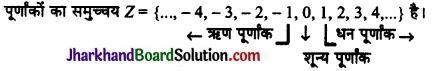(i) संख्या रेखा पर शून्य के दार्यों और के पूर्णांक, धन पूर्णांक तथा शून्य के बायीं ओर के पूर्णांक, ऋण पूर्णांक कहलाते हैं।
(ii) शून्य, प्रत्येक धन पूर्णांक से छोटा तथा सभी ऋण पूर्णांकों से बड़ा होता है।परिमेय संख्याएँ : ऐसी संख्याएँ जो $$\frac{p}{q}$$ के रूप में व्यक्त की जा सकती हों तथा जहाँ p और पूर्णांक हों, परन्तु q ≠ 0, परिमेय संख्याएँ कहलाती हैं।
जैसे : $$\frac{1}{2}, \frac{2}{3}, \frac{-4}{5}, \frac{7}{3}, \frac{-6}{7}, \ldots$$ इत्यादि।

• प्रत्येक प्राकृत संख्या परिमेय संख्या है।
• 0 एक परिमेय संख्या है।
• प्रत्येक पूर्णांक परिमेय संख्या है।
• प्रत्येक भिन्न परिमेय संख्या है।
• प्रत्येक परिमेय संख्या का दशमलव भिन्न के रूप में प्रसार किया जा सकता है।

परिमेय संख्याओं का दशमलव प्रसार दो प्रकार का होता है:
(i) सांत,
(ii) असांत (अनवसानी आवर्ती)।

सांत दशमलव प्रसार : परिमेय संख्याएँ, जो कि सदा $$\frac{p}{q}$$ के रूप में होती है, p में q का भाग देने पर अन्त में शेषफल शुन्य प्राप्त होता है, तो वे दशमलव संख्याएँ सांत दशमलव प्रसार वाली परिमेय संख्याएँ कहलाती हैं।
जैसे : $$\frac{1}{2}$$ = 0.5, $$\frac{3}{2}$$ = 1.5, $$\frac{1}{8}$$ = 0.125 आदि।

असांत दशमलव प्रसार : परिमेय संख्या को दशमलव संख्या में बदलते समय भाग की क्रिया निरन्तर चलती रहती है और शेषफल की निरंतरता बनी रहती है अर्थात् भागफल में अंकों की पुनरावृत्ति होती रहती है इस प्रकार की दशमलव संख्याओं को असांत आवर्ती दशमलव संख्या कहते हैं असांत दशमलव संख्या को संक्षिप्त रूप में लिखने के लिए पुनरावृत्ति वाले अंकों के ऊपर (-) रेखा खींचते हैं।
जैसे : $$\frac{3}{11}$$ = 0.272727…….. = $$0 . \overline{27}$$
$$\frac{1}{3}$$ = 0.333……. = $$0 . \overline{3}$$ इत्यादि।
इन्हें असांत आवर्ती (Non-terminating Repeating) परिमेय संख्याएँ भी कहते हैं।परिमेय संख्याओं के महत्त्वपूर्ण बिन्दु :

1. प्राकृत संख्याएँ, पूर्ण संख्याएँ, पूर्णांक व भिन्नें सभी परिमेय संख्याएँ होती हैं।
2. भिन्न के अंश और हर प्राकृत संख्याएँ होती हैं, जबकि परिमेय संख्या के अंश और हर पूर्णांक होते हैं। परन्तु परिमेय संख्या का हर शून्य नहीं होता।
3. संख्या रेखा पर परिमेय संख्याएँ प्रदर्शित की जा सकती हैं।
4. किसी परिमेय संख्या के अंश और हर को समान अशून्य पूर्णांक से गुणा करने अथवा भाग देने पर समतुल्य परिमेय संख्याएँ प्राप्त होती हैं।
5. दो परिमेय संख्याओं के बीच में अनन्त परिमेय संख्याएँ निहित होती हैं।
6. प्रत्येक परिमेय संख्या को सांत अथवा असांत आवर्ती दशमलव संख्या के रूप में लिखा जा सकता है।
7. सांत परिमेय संख्या का हर सदैव 2m × 5n के रूप में होता है।
8. यदि परिमेय संख्या का हर 2m × 5n के रूप में नहीं है तो वह असांत आवृत्ति होती है।
9. यदि परिमेय संख्या के अंश तथा हर में एक के अतिरिक्त अन्य कोई उभयनिष्ठ गुणनखण्ड नहीं है तो वह परिमेय संख्या का मानक रूप (Standard form) कहलाता है।
10. परिमेय संख्या ऋणात्मक हो सकती है किन्तु उसका हर सदैव धनात्मक ही लिया जाता है।

## JAC Jharkhand Board Class 11th Geography Important Questions in Hindi & English Medium

### JAC Board Class 11th Geography Important Questions in Hindi Medium

Jharkhand Board Class 11th Geography Important Questions: भौतिक भूगोल के मूल सिद्धांत

Jharkhand Board Class 11th Geography Important Questions: भारत : भौतिक पर्यावरण

### JAC Board Class 11th Geography Important Questions in English Medium

JAC Board Class 11th Geography Important Questions: Fundamentals of Physical Geography

• Chapter 1 Geography as a Discipline Important Questions
• Chapter 2 The Origin and Evolution of the Earth Important Questions
• Chapter 3 Interior of the Earth Important Questions
• Chapter 4 Distribution of Oceans and Continents Important Questions
• Chapter 5 Minerals and Rocks Important Questions
• Chapter 6 Geomorphic Processes Important Questions
• Chapter 7 Landforms and their Evolution Important Questions
• Chapter 8 Composition and Structure of Atmosphere Important Questions
• Chapter 9 Solar Radiation, Heat Balance and Temperature Important Questions
• Chapter 10 Atmospheric Circulation and Weather Systems Important Questions
• Chapter 11 Water in the Atmosphere Important Questions
• Chapter 12 World Climate and Climate Change Important Questions
• Chapter 13 Water (Oceans) Important Questions
• Chapter 14 Movements of Ocean Water Important Questions
• Chapter 15 Life on the Earth Important Questions
• Chapter 16 Biodiversity and Conservation Important Questions

JAC Board Class 11th Geography Important Questions: India Physical Environment

• Chapter 1 India – Location Important Questions
• Chapter 2 Structure and Physiography Important Questions
• Chapter 3 Drainage System Important Questions
• Chapter 4 Climate Important Questions
• Chapter 5 Natural Vegetation Important Questions
• Chapter 6 Soils Important Questions
• Chapter 7 Natural Hazards and Disasters Important Questions

## JAC Jharkhand Board Class 11th Geography Solutions in Hindi & English Medium

### JAC Board Class 11th Geography Solutions in Hindi Medium

Jharkhand Board Class 11th Geography Part 1 Fundamentals of Physical Geography (भौतिक भूगोल के मूल सिद्धांत भाग-1)

Jharkhand Board Class 11th Geography Part 2 India Physical Environment (भारत : भौतिक पर्यावरण भाग-2)

### JAC Board Class 11th Geography Solutions in English Medium

JAC Board Class 11th Geography Part 1 Fundamentals of Physical Geography

• Chapter 1 Geography as a Discipline
• Chapter 2 The Origin and Evolution of the Earth
• Chapter 3 Interior of the Earth
• Chapter 4 Distribution of Oceans and Continents
• Chapter 5 Minerals and Rocks
• Chapter 6 Geomorphic Processes
• Chapter 7 Landforms and their Evolution
• Chapter 8 Composition and Structure of Atmosphere
• Chapter 9 Solar Radiation, Heat Balance and Temperature
• Chapter 10 Atmospheric Circulation and Weather Systems
• Chapter 11 Water in the Atmosphere
• Chapter 12 World Climate and Climate Change
• Chapter 13 Water (Oceans)
• Chapter 14 Movements of Ocean Water
• Chapter 15 Life on the Earth
• Chapter 16 Biodiversity and Conservation

JAC Board Class 11th Geography Part 2 India Physical Environment

• Chapter 1 India – Location
• Chapter 2 Structure and Physiography
• Chapter 3 Drainage System
• Chapter 4 Climate
• Chapter 5 Natural Vegetation
• Chapter 6 Soils
• Chapter 7 Natural Hazards and Disasters

## JAC Class 9 Maths Notes Chapter 10 Circles

Students should go through these JAC Class 9 Maths Notes Chapter 10 Circles will seemingly help to get a clear insight into all the important concepts.

### JAC Board Class 9 Maths Notes Chapter 10 Circles

Definitions
→ Circle: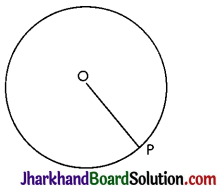The collection of all the points in a plane, which are at a fixed distance from a fixed point in the plane, is called a circle. The fixed point is called the centre of the circle and the fixed distance is called the radius of the circle.
In figure, O is the centre and the length OP is the radius of the circle. So the line segment joining the centre and any point on the circle is called a radius of the circle.

→ Interior and Exterior of a Circle: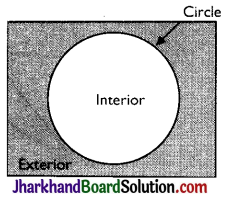A circle divides the plane on which it lies into three parts. They are
(i) inside the circle (or interior of the circle)
(ii) the circle and
(iii) outside the circle (or exterior of the circle.)
The circle and its interior make up the circular region.

→ Chord:
If we take two points P and Q on a circle, then the line segment PQ is called a chord of the circle.→ Diameter: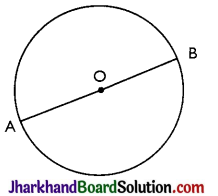The chord which passes through the centre of the circle, is called a diameter of the circle.
A diameter is the longest chord and all diameters have the same length, which is equal to two times the radius. In figure, AOB is a diameter of circle.

→ Arc:
A piece of a circle between two points is called an arc. If we look at the pieces of the circle between two points Pand Q in figure, we find that there are two pieces, one longer and the other smaller. The longer one is called the major arc PQ and the shorter one is called the minor arc PQ. The minor arc PQ is also denoted by PQ and the major arc PQ by PRO, where is some point on the are between P and Q. Unless otherwise stated, arc PQ or PQ stands for minor arc PQ. When P and Q are ends of a diameter, then both arcs are equal and each is called a semicircle.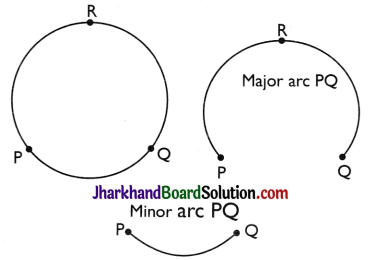→ Circumference: The length of the complete circle is called its circumference.

→ Segment: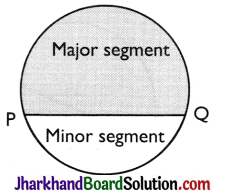The region between a chord and either of its arcs is called a segment of the circular region or simply a segment of the circle. There are two types of segments which are the major segment and the minor segment (as in figure).

→ Sector:
The region between an arc and the two radil, joining the centre to the end points of the arc is called a sector. Like segments, we find that the minor arc corresponds to the minor sector and the major arc corresponds to the major sector. In figure, the region OPQ is the minor sector and the remaining part of the circular region is the major sector. When two arcs are equal, then both segments and both sectors become the same and each is known as a semicircular region.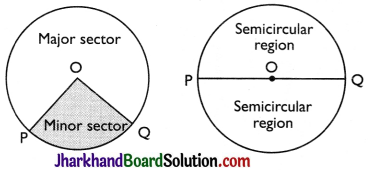Theorem 1.
Equal chords of a circle subtend equal angles at the centre.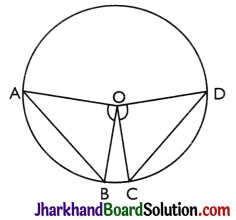Given: AB and CD are the two equal chords of a circle with centre O.
To Prove: ∠AOB = ∠COD.
Proof:
In ΔAOB and ΔCOD,
OA = OC [Radii of a circle]
OB = OD [Radii of a circle]
AB = CD [Given]
∴ ΔAOB ≅ ΔCOD [By SSS]
∴ ∠AOB = ∠COD. [By CPCT]

Converse of above Theorem:
If the angles subtended by the chords of a circle at the centre are equal, then the chords are equal.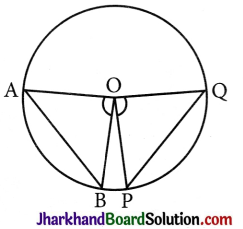Given: ∠AOB and ∠POQ are two equal angles subtended by chords AB and PQ of a circle at its centre O.
To Prove: AB = PQ
Proof:
In ΔAOB and ΔPOQ.
OA = OP [Radii of a circle]
OB = OQ [Radii of a circle]
∠AOB = ∠POQ [Given]
∴ ΔAOB ≅ ΔPOQ [By SAS]
∴ AB = PQ [By CPCT]
Hence, proved.Theorem 2.
The perpendicular from the centre of a circle to a chord bisects the chord.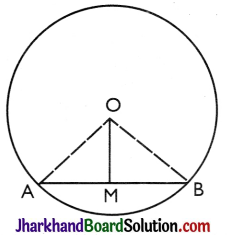Given: A circle with centre O. AB is a chord of this circle OM ⊥ AB.
To Prove: MA = MB.
Construction: Join OA and OB.
Proof:
In right triangles OMA and OMB.
OA = OB [Radii of a circle]
OM = OM [Common]
∠OMA = ∠OMB [90° each]
∴ ΔOMA ≅ ΔOMB [By RHS]
∴ MA = BR [By CPCT]
Hence, proved.

Converse of above Theorem:
The line drawn through the centre of a circle to bisect a chord is a perpendicular to the chord.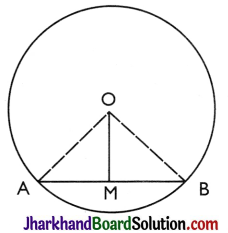Given: A circle with centre O. AB is a chord of this circle whose mid-point is M.
To Prove: OM ⊥ AB
Construction: Join OA and OB.
Proof:
In ΔOMA and ΔOMB,
MA = MB [Given]
OM = OM [Common]3
ОА = ОВ [Radii of a circle]
∴ ΔOMA ≅ ΔOMB [By SSS]
∴ ∠AMO = ∠BMO [By CPCT]
But, ∠AMO + ∠BMO = 180° [Linear pair axiom]
∴ ∠AMO = ∠BMO = 90°
⇒ OM ⊥ AB.

Theorem 3.
There is one and only one circle passing through three given non-collinear points.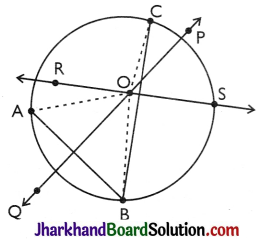Proof:
Let us take three points A, B and C, which are not on the same line, or in other words, they are not collinear [as in figure]. Draw perpendicular bisectors of AB and BC say, PQ and RS respectively. Let these perpendicular bisectors intersect at one point O. (Note that PQ and RS will intersect because they are not parallel) (as in figure).
∴ Olies on the perpendicular bisector PQ of AB.
∴ OA = OB
[ Every point on the perpendicular bisector of a line segment is equidistant from its end points]
∴ Similarly : Olies on the perpendicular bisector RS of BC.
∴ OB = OC
[∵ Every point on the perpendicular bisector of a line segment is equidistant from its end points]
So, OA = OB = OC
i.e., the points A, B and C are at equal distances from the point O.

So, if we draw a circle with centre O and radius OA it will also pass through B and C. This shows that there is a circle passing through the three points A, B and C. We know that two lines (perpendicular bisectors) can intersect at only one point. so we can draw only one circle with radius OA. In other words, there is a unique circle passing through A, B and C. Hence Proved.

Remark:
If ABC is a triangle, then by above theorem, there is a unique circle passing through the three vertices A, B and C of the triangle. This circle is the circumcircle of the ΔABC. Its centre and radius are called respectively the circumcentre and the circumradius of the triangle.Theorem 4.
Equal chords of a circle (or of congruent circles) are equidistant from the centre (or centres).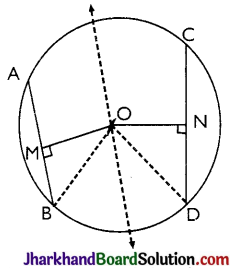Given: A circle has two equalchords AB and CD.
So, AB = CD and OM ⊥ AB, ON ⊥ CD.
To Prove: OM = ON
Construction: Join OB and OD.
Proof:
AB = CD (Given)
∴ $$\frac{1}{2}$$AB = $$\frac{1}{2}$$CD
⇒ BM = DN
[∵ The perpendicular drawn from the centre of a circle to the chord bisect the chord.]
In ΔΟΜB and ΔOND,
∠OMB = ∠OND = 90° [Given]
OB = OD [Radii of same circle]
BM = DN [Proved above]
∴ ΔOMB ≅ ΔOND [By RHS]
OM = ON [By CPCT]
Hence, proved.

Remark: Chords equidistant from the centre of a circle are equal in length.

Some Important Theorems

Theorem 1.
If the angles subtended by the chords at the centre of a circleare equal then the chords are equal.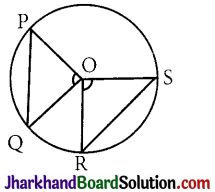Given: A circle with centre O. Chords PQ and RS subtend equal angles at the center of the circle.
i.e. ∠POQ = ∠ROS
To Prove: Chord PQ = chord RS.
Proof:
In ΔPOQ and ΔROS
∠POQ = ∠ROS [Given]
OP = OR [Radii of the same circle]
OQ = OS [Radii of the same circle]
⇒ ΔPOQ ≅ ΔROS [By SAS]
⇒ chord PQ = chord RS [By CPCT]
Hence, proved.

Corollary 1: Two ares of a circle are congruent, if the angles subtended by them at the centre are equal.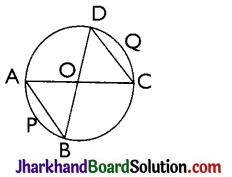Corollary 2: If two arcs of a circle are equal, they subtend equal angles at the centre.
Corollary 3: If two arcs of a circle are congruent (equal), their corresponding chords are also equal. Conversely, if two chords of a circle are equal, their corresponding arcs are also equal
∠AOB = ∠COD
∴ Chord AB = Chord CD
∴ Arc APB = Arc CQD.Theorem 2.
The angle subtended by an arc at the centre is double the angle subtended by it at any point on the remaining part of the circle.
Given: An arc PQ of a circle subtending angles POQ at the centre and PAQ at a point A on the remaining part of the circle.
To Prove: ∠POQ = 2∠PAQ.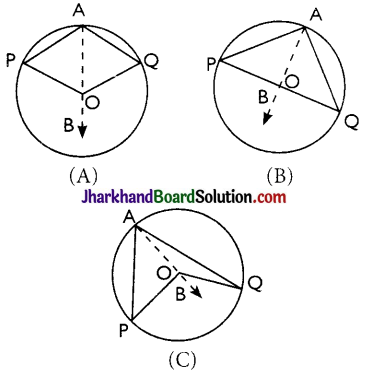Construction: Join AO and extend it to a point B.
Proof:
There arises three cases:
(A) arc PQ is minor
(B) arc PQ is a semi-circle
(C) arc PQ is major.
In all the cases,
∠BOQ = ∠OAQ + ∠AQO ….(i)
[∵ An exterior angle of triangle is equal to the sum of the two interior opposite angles]
In OAO,
OA = OQ [Radil of a circle]
∠OAQ = ∠OQA …..(ii)
[Angles opposite to equal sides of a triangle are equal]
From (i) and (ii),
∠BOQ = 2∠OAQ ……(iii)
Similarly,
∠BOP = 2∠OAP ……(iv)
Adding (i) and (iv), we get
∠BOP + ∠BOQ = 2(∠OAP + ∠OAQ)
⇒ ∠POQ = 2∠PAQ ….(v)
NOTE: For the case (C), where PQ is the major arc, angle is replaced by reflex angles
Thus, ∠POQ = 2∠PAQ.

Theorem 3.
Angles in the same segment of a circle are equal.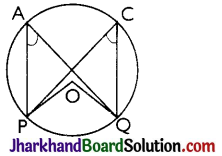Proof:
Let P and Q be any two points on a circle to form a chord PQ, A and C any other points on the remaining part of the circle and O be the centre of the circle. Then,
∠POQ = 2∠PAQ ……(i)
And ∠POQ = 2∠PCQ ……(ii)
From above equations, we get
2∠PAQ = 2∠PCQ
⇒ ∠PAQ = ∠PCQ Hence, provedTheorem 4.
Angle in the semicircle is a right angle.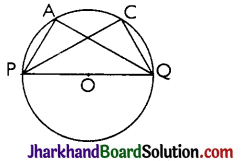Proof:
∠PAQ is an angle in the segment, which is a semicircle.
∴ ∠PAQ = $$\frac{1}{2}$$∠POQ = $$\frac{1}{2}$$ × 180° = 90°
[∵ ∠POQ is straight line angle or ∠POQ = 180°]
If we take any other point C on the semicircle then again we get
∠PCQ = $$\frac{1}{2}$$∠POQ = $$\frac{1}{2}$$ × 180° = 90°
Hence, proved.

Theorem 5.
If a line segment joining two points subtends equal angles at two other points lying on the same side of the line segment then the four points lie on a circle (i.e., they are concyclic).
Given: AB is a line segment, which subtends equal angles at two points C and D i.e, ∠ACB = ∠ADB.
To Prove: The points A, B, C and D lie on a circle.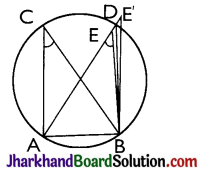Proof:
Let us draw a circle through the points A, C and B. Suppose it does not pass through the point D.
Then it will intersect AD (or extended AD) at a point, say E (or E’). If points A, C, E and B lie on a circle.
∠ACB = ∠AEB [∵ Angles in the same segment of circle are equal]
But it is given that, ∠ACB = ∠ADB
This is possibleonly when Ecoincides with D.
Similarly, E’ should also coincide with D.
So A, B, C and D are concyclic.
Hence, proved.

A quadrilateral ABCD is called cyclic if all the four vertices of it lie on a circle.Theorem 6.
The sum of either pair of opposite angles of a cyclic quadrilateral is 180°.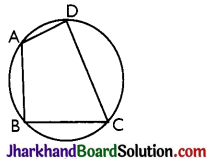To Prove: ∠A + ∠C = ∠B + ∠D = 180°
Construction: Join AC and BD.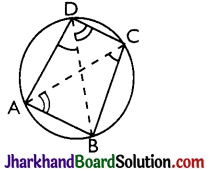Proof:
∠ACB = ∠ADB [Angles in the same segment]
And ∠BAC = ∠BDC [Angles in the same segment]
∴ ∠ACB + ∠BAC = ∠ADB + ∠BDC
Adding ∠ABC to both sides, we get
∠ACB + ∠BAC + ∠ABC = ∠ADC + ∠ABC
The left side being the sum of three angles of AABC is equal to 180°.
∴ ∠ADC + ∠ABC = 180°
i.e, ∠D + ∠B = 180°
∴ ∠A + ∠C = 360° – (∠B + ∠D) = 180°
[∵ ∠A + ∠B + ∠C + ∠D = 360°]
Hence, proved.
Corollary: If the sum of a pair of opposite angles of a quadrilateral is 180°, then quadrilateral is cyclic.

## JAC Class 9 Maths Notes Chapter 13 Surface Areas and Volumes

Students should go through these JAC Class 9 Maths Notes Chapter 13 Surface Areas and Volumes will seemingly help to get a clear insight into all the important concepts.

### JAC Board Class 9 Maths Notes Chapter 13 Surface Areas and Volumes

Solid Figures:
If any figure such as cuboids, has three dimensions length, width and height then it is known as three dimensional figures. Rectangle has only two dimensions i.e. length and width. Three dimensional figures have volume in addition to areas of surface from which these solid figures are formed.

→ Cuboid:
There are six faces (rectangular), eight vertices and twelve edges in a cuboid.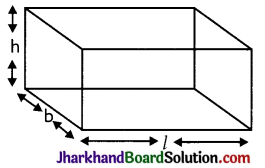Total Surface Area (T.S.A.): The area of surface from which cuboid is formed. If l, b, h be length, breadth and height of a cuboid respectively then
(i) Total Surface Area (T.S.A.) = 2 [l × b + b × h + h × l]
(ii) Lateral Surface Area (L.S.A.) = 2h (b + l)
(or Area of 4 walls) = 2h(l + b)
(iii) Volume of Cuboid = (Area of base) × height = (l × b) × h
(iv) Length of diagonal = $$\sqrt{l^2+b^2+h^2}$$

→ Cube:
Cube has six faces. Each face is a square.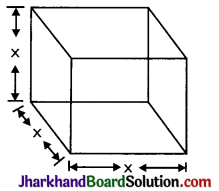If x is a side of cube, then
(i) T.S.A. = 2[x·x + x·x + x·x]
= 2[x2 + x2 + x2] = 2(3x2) = 6x2
(ii) L.S.A. = 2[x2 + x2] = 4x2
(iii) Length of diagonal = x$$\sqrt{3}$$
(iv) Volume = (Area of base) × Height
= (x2) × x = x3

→ Cylinder:
Curved surface area of cylinder (C.S.A.): It is the area of surface from which the cylinder is formed. When we cut this cylinder, we will find a rectangle with length 2πr and height h units.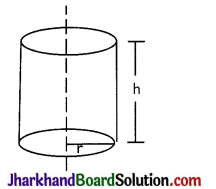If r be the radius and h be height of a cylinder, then
(i) C.S.A. of cylinder = (2πr) × h
= 2πrh.
(ii) T.S.A.C.S.A. + area of circular top and bottom
= 2πrh+ (πr2) + (πr2)
= 2πrh + 2πr2 = 2πr(h + r) sq.units
(iii) Volume of cylinder
= Area of base × height
= (πr2) × h = πr2h cubic units

→ Hollow cylinder: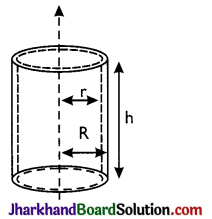(i) C.S.A. of hollow cylinder
= 2π(R + r)h sq. units
(ii) T.S.A. of hollow cylinder
= 2π(R + r)h + 2π(R2 – r2)
= 2π(R + r) [h + R – r] sq. units
(iii) Volume of hollow cylinder
= π(R2 – r2)h cubic units
Where, r = inner radius of cylinder
R = outer radius of cylinder
h = height of the cylinder

→ Cone: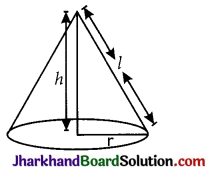(i) C.S.A. of cone = πrl
(ii) T.S.A.of cone = C.S.A. + Base area
πrl + πr2 = πr(l + r)
(ii) Volume of cone = $$\frac{1}{3}$$πr2h
Where, h = height of cone
r = radius of base of cone
l = slant height of cone

→ Sphere: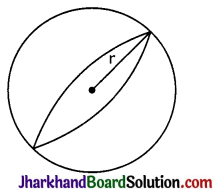(i) T.S.A. of sphere = 4πr2
(ii) Volume of sphere = $$\frac{4}{3}$$πr3
Where r be the radius of the sphere.

→ Hemisphere: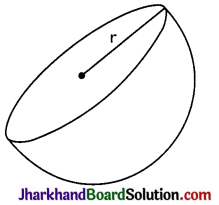(i) C.S.A. = 2πr2
(ii) T.S.A. = C.S.A. + base area
= 2πr2 + πr2 = 3πr2
(iii) Volume = $$\frac{2}{3}$$πr3
Where r is the radius of Hemisphere

→ Hollow Hemisphere: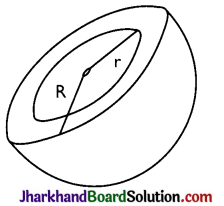(i) C.S.A. = 2π(R2 + r2)
(ii) T.S.A. = 2π(R2 + r2) + π(R2 – r2)
(iii) Volume = $$\frac{2}{3}$$π(R3 – r3)

## JAC Class 9 Maths Notes Chapter 12 Heron’s Formula

Students should go through these JAC Class 9 Maths Notes Chapter 12 Heron’s Formula will seemingly help to get a clear insight into all the important concepts.

### JAC Board Class 9 Maths Notes Chapter 12 Heron’s Formula

Mensuration:
A branch of mathematics which concerns itself with measurement of lengths, areas and volumes of plane and solid figure is called Mensuration.
→ Perimeter: The perimeter of a plane figure is the length of its boundary. In case of a triangle or a polygon, the perimeter is the sum of the lengths of its sides.

→ Units of Perimeter: The unit of perimeter is the same as the unit of length i.e. centimetre (cm), metre (m), kilometre (km) etc.
1 centimetre (cm) = 10 milimetres (mm)
1 decimetre (dm) = 10 centimetres
1 metre (m) = 10 decimetres
= 100 centimetres
= 1000 milimetres
1 decametre (dam) = 10 metres
= 1000 centimetres
1 hectometre (hm) = 10 decametres
= 100 metres
1 kilometre (km) = 1000 metres
= 100 decametres
= 10 hectometresArea:
The area of a plane figure is the measure of the surface enclosed by its boundary.
The area of a triangle or a polygon is the measure of the surface enclosed by its sides.
→ Units of Area:
The various units of measuring area are, square centimetre (cm2), square metre (m2), hectare etc.
1 square centrimetre (cm2)
= 1 cm × 1 cm
= 10 mm × 10 mm
= 100 sq.mm.
1 square decimetre (dm2)
= 1 dm × dm
= 10 cm × 10 cm
= 100 sq. cm.
1 square metre (m2)
= 1 m × 1 m
= 10 dm × 10 dm
= 100 sq. dm
1 square decametre (dam2)
= 1 dam × 1 dam
= 10 m × 10 m
= 100 sq.m.
1 square hectometre (hm2)
= 10 dam × 10 dam
= 100 sq. dam
(or 1 hectare) = 10000 sq. m.
1 square kilometre (km2)
= 1 km × 1 km
= 10 hm × 10 hm
= 100 sq. hm.

→ Heron’s formula: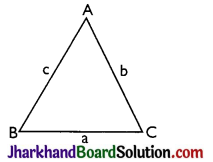In ΔABC if sides of triangle BC, CA, and AB are a, b, c respectively then
perimeter = 2s = a + b + c
Area = $$\sqrt{s(s-a)(s-b)(s-c)}$$

→ Perimeter and Area of a Triangle:
Right-angled triangle: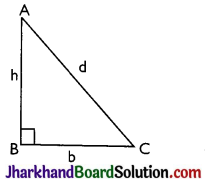For a right-angled triangle, let b be the base, h be the perpendicular and d be the hypotenuse. Then
(A) Perimeter = b + h + d
(B) Area = $$\frac{1}{2}$$(Base × Height) = $$\frac{1}{2}$$bh
(C) Hypotenuse, d = $$\sqrt{b^2+h^2}$$ [Pythagoras theorem]

Isosceles right-angled triangle: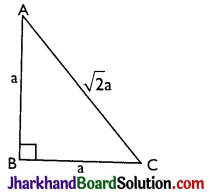For an isosceles right-angled triangle, let a be the equal sides, then
(A) Hypotenuse = $$\sqrt{a^2+a^2}=\sqrt{2} a$$
(B) Perimeter = 2a + $$\sqrt{2}$$a
(C) Area = $$\frac{1}{2}$$(Base × Height) = $$\frac{1}{2}$$(a × a) = $$\frac{1}{2}$$a2.

Equilateral triangle: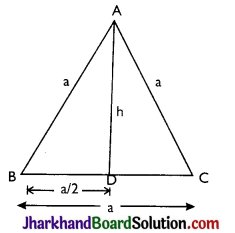For an equilateral triangle, let each side be a, and the height of the triangle be h, then
(A) ∠A = ∠B = ∠C = 60°
(C) AB = BC = AC = a(say)
(D) BD = DC = $$\frac{\mathrm{a}}{2}$$
(E) $$\left(\frac{\mathrm{a}}{2}\right)^2$$ + h2 = a2 ⇒ h2 = $$\frac{3 \mathrm{a}^2}{4}$$
∴ Height (h) = $$\frac{\sqrt{3}}{2} \mathrm{a}$$
(F) Area = $$\frac{1}{2}$$(Base × Height)
= $$\frac{1}{2} \times \mathrm{a} \times \frac{\sqrt{3}}{2} \mathrm{a}=\frac{\sqrt{3}}{4} \mathrm{a}^2$$
(G) Perimeter = a + a + a = 3a.

## JAC Class 9 Maths Notes Chapter 11 Constructions

Students should go through these JAC Class 9 Maths Notes Chapter 11 Constructions will seemingly help to get a clear insight into all the important concepts.

### JAC Board Class 9 Maths Notes Chapter 11 Constructions

To Construct The Bisector Of A Line Segment
Example 1.
Draw a line segment of length 7.8 cm and draw the perpendicular bisector of this line segment.
Solution:
Given: Let the given line segment be AB = 7.8 cm
Steps:

• Draw the line segment AB = 7.8 cm,
• With point A as centre and a suitable radius, more than half the length of AB, draw arcs on both the sides of AB.
• With point B as centre and with the same radius draw arcs on both the sides of AB. Let these arcs cut at points P and Q as shown in the figure.
• Draw a line through the points P and Q. The line so obtained is the required perpendicular bisector of given line segment AB.

Line PQ is perpendicular bisector of AB.
(A) PQ bisects AB i.e., OA = OB.
(B) PQ is perpendicular to AB ie, ∠POA = ∠POB = 90°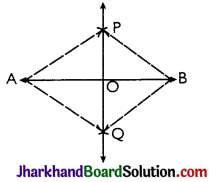Proof: In ΔAPQ and ΔBPQ,
AP = AQ [By construction]
BP = BQ [By construction]
PQ = PQ [Common]
⇒ ΔAPQ ≅ ΔBPQ [By SSS]
⇒ ∠APQ = ∠BPQ [By CPCT]
Now, in ΔAPO and ΔBPO,
AP = BP [By construction]
OP = OP [Common side]
∠APO = ∠BPO [Proved above]
⇒ ΔAPO ≅ ΔBPO [By SAS]
∠POA = ∠POB [CPCT]
and OA = OB
⇒ ∠POA = ∠POB = $$\frac{180^{\circ}}{2}$$ = 90°
[∵∠POA + ∠POB = 180°]
⇒ PQ is perpendicular bisector of AB.To Construct The Bisector Of A Given Angle
Let ABC be the given angle to be bisected.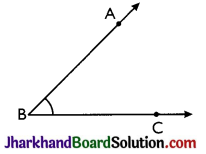Steps:

• With Bas centre and a suitable radius, draw an arc which cuts ray BA at point D and ray BC at point E.
• Taking D and E as centres and with equal radii draw arcs which intersect each other at point E In this step, each equal radius must be more than half the length of arc DE.
• Join B and F and produce to get the ray BF. Ray BF is the required bisector of the given angle ABC.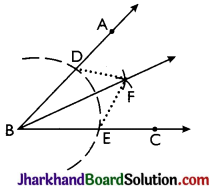Proof:
Join DF and EF.
In ΔBDF and ΔBEF
BD = BE [Radii of the same arc]
DF = EF [Radii of the equal arcs]
BF = BF [Common]
⇒ ΔBDF ≅ ΔBEF [By SSS]
⇒ ∠DBF = ∠EBF [By CPCT]
i.e., ∠ABF = ∠CBF
⇒ BF bisects ∠ABC. Hence, proved.

To Construct The Required Angles
→ To Construct the Required Angle of 60°: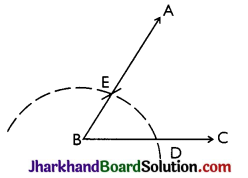Steps:

• Draw the ray BC.
• With B as centre and any suitable radius. draw an arc which cuts BC at point D.
• With D as centre and same radius, as taken in step (ii), draw one more arc which cuts previous arc at point E.
• Join BE and produce up to any point A.
Then, ∠ABC = 60°.

→ To Construct an Angle of 120°: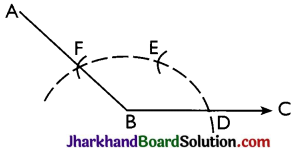Steps:

• Draw the ray BC.
• Taking B as centre and with any suitable radius, draw an arc which cuts BC at point D.
• Taking Das centre, draw an arc of the same radius, as taken in step (ii), which cuts the first arc at point E.
• Taking E as centre and same radius, as taken in step (ii), draw one more arc which cuts the first arc at point E.
• Join BF and produce up to any suitable point A. Then, ∠ABC = 120°.

→ To Construct an Angle of 30°: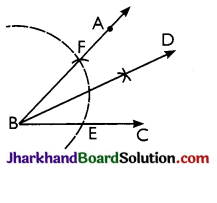Steps:

• Construct angle ABC = 60° by compass.
• Draw BD, the bisector of angle ABC.
Then, ∠DBC = 30°

→ To Construct an Angle of 90°:
Steps: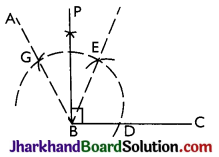• Construct angle ABC = 120° by using compass.
• Draw BP, the bisector of angle EBG.
Then, ∠PBC = 90°

→ To Construct an Angle of 45°: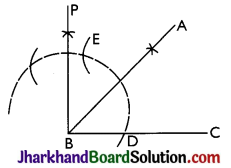Steps:

• Draw ∠PBC = 90° by compass.
• Draw BA which bisects angle PBC,
Then, ∠ABC = 45°.

→ To Construct an Angle of 105°: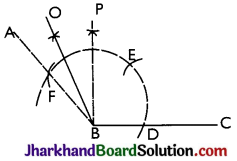Steps:

• Construct ∠ABC = 120° and ∠PBC = 90° by compass.
• Draw BO, the bisector of ∠ABP.
Then, ∠OBC = 105°

→ To Construct an Angle of 150°: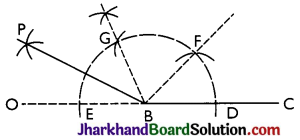Steps:

• Draw the ray BC. Produce CB towards B up to any point.
With B as centre, draw an arc (with any suitable radius) which cuts OC at points D and E.
• With D as centre, draw an arc of the same radius, as taken in step (ii), which cuts the first arc at point E.
• With F as centre, draw one more arc of the same radius, taken in step (ii), which cuts the first arc at point G.
• Draw BP, the bisector of angle EBG.

Now ∠FBD = ∠GBF = ∠EBG = 60° and
∠GBP = ∠PBE = 30°
Then, ∠PBC = 150°

→ To Construct an Angle of 135°: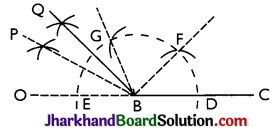Steps:

• Construct ∠PBC = 150° and ∠GBC = 120° by compass.
• Construct BQ, the bisector of angle PBG.
Then, ∠QBC = 135°To Construct A Triangle
Case (i) To construct an equilateral triangle when its one side is given.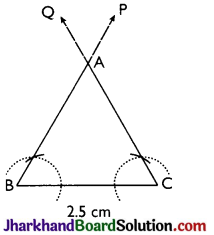Example 2.
Draw an equilateral triangle having each side of 2.5 cm.
Solution:
Given one side of the equilateral triangle be 2.5 cm.
Steps:

• Draw a line segment BC = 2.5 cm.
• Through B, construct ray BP making angle 60° with BC. i.e. ∠PBC = 60°
• Through C, construct ray CQ making angle 60° with BC
i.e., ∠QCB = 60°
• Let BP and CQ intersect each other at point A. Then, AABC is the required equilateral triangle.

Proof:
Since, ∠ABC = ∠ACB = 60°
∠BAC = 180° – (60° +60°) = 60°
⇒ All the angles of the AABC are equal.
⇒ ΔABC is the required equilateral triangle. Hence, proved.

Case (ii) When the base of the triangle, one base angle and the sum of other two sides are given.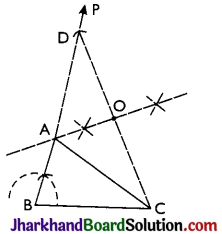Example 3.
Construct a triangle with 3 cm base and sum of other two sides is 8 cm and one base angle is 60°.
Solution:
Given the base BC of the triangle ABC be 3 cm, one base angle ∠B = 60° and the sum of the other two sides be 8 cm
i.e, AB + AC = 8 cm.
Steps:

• Draw BC = 3 cm.
• At point B, draw ray BP so that ∠PBC = 60°.
• From BP, cut BD = 8 cm.
• Join D and C.
• Draw perpendicular bisector of CD, which meets BD at point A.
• Join A and C.

Thus, ABC is the required triangle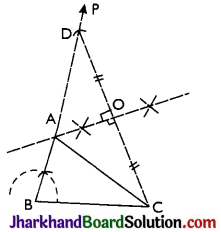Proof: Since, OA is perpendicular bisector of CD
⇒ OC = OD
∠AOC = ∠AOD = 90°
Also, OA = OA [Common]
∴ ΔAOC ≅ ΔAOD [By SAS]
∴ BA + AC = BA + AD = BD = 8cm.

Example 4.
Construct a right triangle, when one side is 3.8 cm and the sum of the other side and hypotenuse is 6 cm.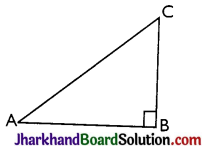Solution:
Here, consider the ΔABC, as shown in figure.
Clearly, AB = 3.8 cm, ∠B = 90° and BC + AC = 6 cm.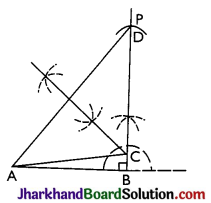Steps:

• Draw AB = 3.8 cm.
• Through B. draw ray BP so that ∠ABP = 90°.
• From BP, cut BD = 6 cm.
• Join A and D.
• Draw perpendicular bisector of AD, which meets BD at point C.
Thus, ABC is the required triangle.

Case (iii) When the base of the triangle, one base angle and the difference of the other two sides are given.

Example 5.
Construct a triangle with base of 8 cm and difference between the length of other two sides is 3 cm and one base angle is 60°.
Solution:
Given the base BC of the required triangle ABC be 8 cm i.e., BC = 8 m, base angle B = 60° and the difference between the lengths of other two sides AB and AC be 3 cm.
i.e, AB – AC = 3 cm or AC – AB = 3 cm.
(a) When AB – AC = 3 cm i.e., AB > AC:
Steps:

• Draw BC = 8 cm.
• Through point B, draw BP so that ∠PBC = 60°.
• From BP cut BD = 3 cm.
• Join D and C.
• Draw perpendicular bisector of DC; which meets BP at point A.
• Join A and C.

Thus, ΔABC is the required triangle.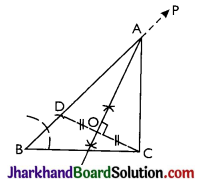Proof:
Since OA is perpendicular bisector of CD.
⇒ OD = OC
∠AOD = ∠AOC = 90°
And, OA = OA [Common]
∴ ΔAOD ≅ ΔAOC [By SAS]
⇒ AD = AC [By CPCT]
Now, AB – AC = AB – AD = BD = 3 cm

(b) When AC – AB = 3 cm i.e, AB < AC:
Steps:

• Draw BC = 8 cm
• Through B, draw line BP so that ∠PBC = 60°
• Produce BP backward up to a suitable point Q.
• From BQ, cut BD = 3 cm.
• Join D and C.
• Draw perpendicular bisector of DC, which meets BP at point A.
• Join A and C.

Thus, ΔABC is the required triangle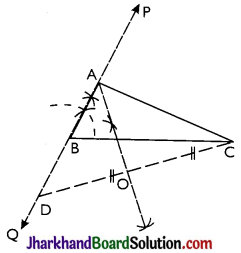Proof:
Since, OA is perpendicular bisector of CD
⇒ OD = OC
∠AOD = ∠AOC = 90°
And OA = OA [Common]
∴ ΔAOD ≅ ΔAOC [By SAS]
⇒ AD = AC [By CPCT]
Now, AC – AB = AD – AB = BD = 3cm

Case (iv) When the perimeter of the triangle and both the base angles are given.Example 6.
Construct a triangle ABC with AB + BC + CA = 12 cm; ∠B = 45° and ∠C = 60°.
Solution:
Given the perimeter of the triangle ABC be 12 cm i.e., AB + BC + CA = 12 cm and both the base angles be 45° and 60° i.e., ∠B = 45° and ∠C = 60°
Steps:

• Draw a line segment PQ = 12 cm.
• At P, construct ray PR so that ∠RPQ = 45° and at Q. construct ray QS so that ∠SQP = 60°.
• Draw bisector of angles RPQ and SQP which meet each other at point A.
• Draw perpendicular bisector of AP, which meets PQ at point B.
• Draw perpendicular bisector of AQ, which meets PQ at point C.
• Join AB and AC.

Thus, ABC is the required triangle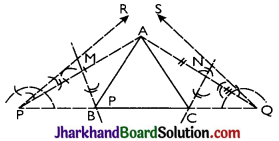Proof:
Since, MB is perpendicular bisector of AP.
⇒ AM = PM
∠AMB = ∠PMB = 90°
BM = BM
⇒ ΔAMB ≅ ΔPMB [By SAS]
PB = AB [By CPCT]
Similarly, NC is perpendicular bisector of AQ.
⇒ ΔQNC ≅ ΔANC [By SAS]
⇒ CQ = AC [By CPCT]
Now, PQ = PB + BC + CQ = AB + BC + AC
= Given perimeter of the AABC drawn.
Also, ∠BPA = ∠BAP [AS ΔPMB ≅ ΔMB]
∴ ∠ABC = ∠BPA + ∠BAP
[Ext. angle of a triangle = sum of two interior opposite angles]
∠ABC = 2∠BPA = ∠RPB = 45°
∠ACB= ∠CQA + ∠CAQ = 2∠CQA
[∵ ΔQNC ≅ ΔANC ∴ ∠CQA = ∠CAQ]
= ∠SQC = 60°

## JAC Class 9 Maths Notes Chapter 6 Lines and Angles

Students should go through these JAC Class 9 Maths Notes Chapter 6 Lines and Angles will seemingly help to get a clear insight into all the important concepts.

### JAC Board Class 9 Maths Notes Chapter 6 Lines and Angles

Line:
A line has length but no width and no thickness.

Angle:
An angle is formed by two non-collinear rays with a common initial point. The common initial point is called the ‘vertex’ of the angle and two rays are called the ‘arms’ of the angle.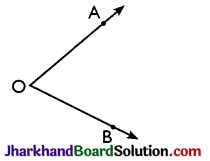Remark:
Every angle has a measure and unit of measurement is degree. One right angle = 90°
1° = 60′ (minutes)
1′ = 60″ (seconds)

Angle addition axiom: If X is a point in the interior of BAC, then
∠BAC = ∠BAX + ∠XAC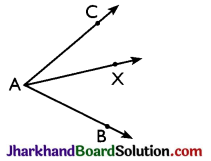Types of Angles:
→ Right angle: An angle whose measure is 90° is called a right angle.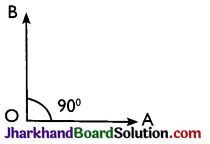→ Acute angle: An angle whose measure is more than 0° but less than 90° is called an acute angle.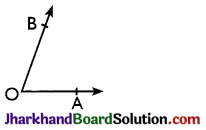0° < ∠BOA < 90°
→ Obtuse angle: An angle whose measure is more than 90° but less than 180° is called an obtuse angle.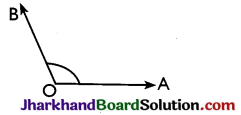90° < ∠AOB < 180°
→ Straight angle: An angle whose measure is 180° is called a straight angle.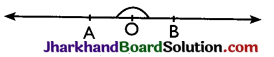→ Reflex angle: An angle whose measure is more than 180° but less than 360 is called a reflex angle.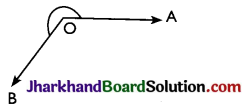180° < ∠AOB < 360°
→ Complementary angles: Two angles whose sum is 90° are called complementary angles.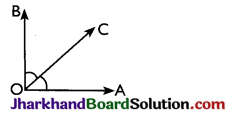∠AOC and ∠BOC are complementary as
∠AOC + ∠BOC = 90°
→ Supplementary angles: Two angles whose sum is 180° are called the supplementary angles.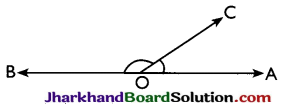∠AOC and ∠BOC are supplementary as their sum is 180°.
→ Angle bisectors: A ray OX is said to be the bisector of ∠AOB, if X is a point in the interior of ∠AOB, and ∠AOX = ∠BOX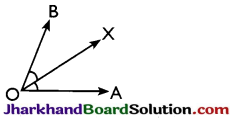→ they have the same vertex,
→ they have a common arm,
→ non-common arms are on either side of the common arm.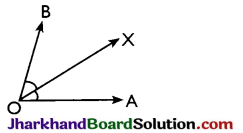∠AOX and ∠BOX are adjacent angles as o is the common vertex, OX is common arm, OA and OB are non-common arms and lie on either side of OX.
→ Lincar pair of angles: Two adjacent angles are said to form a linear pair if their non-common arms are two opposite rays.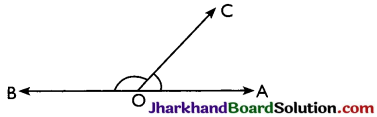→ Vertically opposite angles: Two angles are called a pair of vertically opposite angles, if their arms form two pairs of opposite rays.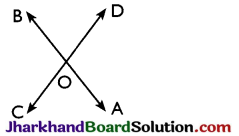∠AOC and ∠BOD form a pair of vertically opposite angles. Also ∠AOD and ∠BOC form a pair of vertically opposite angles.Angles Made by a Transversal with two Parallel Lines:
→ Transversal: A line which intersects two or more given lines at distinct points is called a transversal of the given lines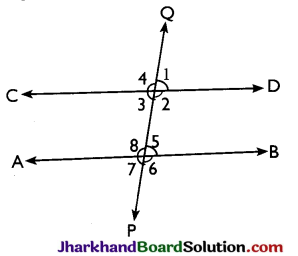→ Corresponding angles: Two angles on the same side of transversal are known as the corresponding angles if both lie either above the two lines or below the two lines, in figure 1 and 5, 4 and 8, 2 and 6, 3 and 7 are the pairs of corresponding angles.
→ Alternate interior angles: ∠3 and ∠5, ∠2 and ∠8, are the pairs of alternate interior angles.
→ Consecutive interior angles: The pair of interior angles on the same side of the transversal are called pair of consecutive interior angles. In figure ∠2 and ∠5, ∠3 and ∠8. are the pairs of consecutive interior angles.
→ Corresponding angles axiom: If a transversal intersects two parallel lines, then each pair of corresponding angles are equal. Conversely, if a transversal intersects two lines, making a pair of equal corresponding angles, then the lines are parallel.

Important Facts to Remember:
→ If a ray stands on line, then the sum of the adjacent angles so formed is 180°.
→ If the sum of two adjacent angles is 180°, then their non-common arms are two apposite rays.
→ The sum of all the angles round a point is equal to 360°.
→ If two lines intersect, then the vertically opposite angles are equal.
→ If a transversal interests two parallel lines then the corresponding angles are equal, each pair of alternate interior angles is equal and each pair of consecutive interior angles is supplementary.
→ If a transversal intersects two lines in such a way that a pair of alternate interior angles are equal, then the two lines are parallel.
→ If a transversal intersects two lines in such a way that a pair of consecutive interior angles are supplementary, then the two lines are parallel.
→ If two parallel lines are intersected by a transversal, the bisectors of any pair of alternate interior angles are parallel and the bisectors of any two corresponding angles are also parallel.
→ If a line is perpendicular to one of the two given parallel lines, then it is also perpendicular to the other line.
→ Two angles which have their arms parallel are either equal or supplementary.
→ Two angles whose arms are perpendicular are either equal or supplementary.

Important Theorems:
Theorem 1.
If two lines intersect each other, then the vertically opposite angles are equal.
Proof:
Given: Two lines AB and CD intersecting at a point O.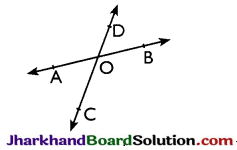To prove: ∠AOC = ∠BOD, ∠BOC
= ZAOD
Proof: Since ray OD stands on AB
again, ray OA stands on CD
⇒ ∠AOD + ∠DOB = 180° [linear pair] ……(i)
⇒ ∠AOC + ∠AOD = 180° [linear pair] ……(ii)
From equations (i) and (ii), we get
∠AOD + ∠DOB
= ∠AOC + ∠AOD
⇒ ∠AOC = ∠DOB
Similarly, we can prove that
∠BOC = ∠DOA Hence, proved.Theorem 2.
If a transversal intersects two parallel lines, then each pair of alternate interior angles is equal.
Proof:
Given: AB and CD are two parallel lines. Transversal l intersects AB and CD at P and Q respectively making two pairs of alternate interior angles, ∠1, ∠2 and ∠3, ∠4.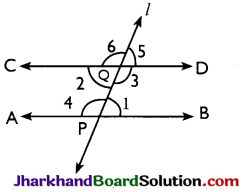To prove: ∠1 = ∠2 and ∠3 = ∠4
Proof: Clearly, ∠2 = ∠5 [Vertically opposite angles]
And, ∠1 = ∠5 [Corresponding angles]
∴ ∠1 = ∠2
Also, ∠3 = ∠6 [Vertically opposite angles]
And, ∠4 = ∠6 [Corresponding angles]
∴ ∠3 = ∠4 Hence, proved.

## JAC Jharkhand Board Class 10th Maths Solutions in Hindi & English Medium

### JAC Board Class 10th Maths Solutions in English Medium

JAC Class 10 Maths Chapter 1 Real Numbers

JAC Class 10 Maths Chapter 2 Polynomials

JAC Class 10 Maths Chapter 3 Pair of Linear Equations in Two Variables

JAC Class 10 Maths Chapter 4 Quadratic Equations

JAC Class 10 Maths Chapter 5 Arithmetic Progressions

JAC Class 10 Maths Chapter 6 Triangles

JAC Class 10 Maths Chapter 7 Coordinate Geometry

JAC Class 10 Maths Chapter 8 Introduction to Trigonometry

JAC Class 10 Maths Chapter 9 Some Applications of Trigonometry

JAC Class 10 Maths Chapter 10 Circles

JAC Class 10 Maths Chapter 11 Constructions

JAC Class 10 Maths Chapter 12 Areas Related to Circles

JAC Class 10 Maths Chapter 13 Surface Areas and Volumes

JAC Class 10 Maths Chapter 14 Statistics

JAC Class 10 Maths Chapter 15 Probability

### JAC Board Class 10th Maths Solutions in Hindi Medium

JAC Class 10 Maths Chapter 1 वास्तविक संख्याएँ

JAC Class 10 Maths Chapter 2 बहुपद

JAC Class 10 Maths Chapter 3 दो चरों वाले रैखिक समीकरण युग्म

JAC Class 10 Maths Chapter 4 द्विघात समीकरण

JAC Class 10 Maths Chapter 5 समांतर श्रेढ़ियाँ

JAC Class 10 Maths Chapter 6 त्रिभुज

JAC Class 10 Maths Chapter 7 निर्देशांक ज्यामिति

JAC Class 10 Maths Chapter 8 त्रिकोणमिति का परिचय

JAC Class 10 Maths Chapter 9 त्रिकोणमिति के कुछ अनुप्रयोग

JAC Class 10 Maths Chapter 10 वृत्त

JAC Class 10 Maths Chapter 11 रचनाएँ

JAC Class 10 Maths Chapter 12 वृतों से संबंधित क्षेत्रफल

JAC Class 10 Maths Chapter 13 पृष्ठीय क्षेत्रफल एवं आयतन

JAC Class 10 Maths Chapter 14 सांख्यिकी

JAC Class 10 Maths Chapter 15 प्रायिकता

## JAC Jharkhand Board Class 12th Maths Solutions in Hindi & English Medium

### JAC Board Class 12th Maths Solutions in English Medium

JAC Class 12 Maths Chapter 1 Relations and Functions

• Chapter 1 Relations and Functions Ex 1.1
• Chapter 1 Relations and Functions Ex 1.2
• Chapter 1 Relations and Functions Ex 1.3
• Chapter 1 Relations and Functions Ex 1.4
• Chapter 1 Relations and Functions Miscellaneous Exercise

JAC Class 12 Maths Chapter 2 Inverse Trigonometric Functions

• Chapter 2 Inverse Trigonometric Functions Ex 2.1
• Chapter 2 Inverse Trigonometric Functions Ex 2.2
• Chapter 2 Inverse Trigonometric Functions Miscellaneous Exercise

JAC Class 12 Maths Chapter 3 Matrices

• Chapter 3 Matrices Ex 3.1
• Chapter 3 Matrices Ex 3.2
• Chapter 3 Matrices Ex 3.3
• Chapter 3 Matrices Ex 3.4
• Chapter 3 Matrices Miscellaneous Exercise

JAC Class 12 Maths Chapter 4 Determinants

• Chapter 4 Determinants Ex 4.1
• Chapter 4 Determinants Ex 4.2
• Chapter 4 Determinants Ex 4.3
• Chapter 4 Determinants Ex 4.4
• Chapter 4 Determinants Ex 4.5
• Chapter 4 Determinants Ex 4.6
• Chapter 4 Determinants Miscellaneous Exercise

JAC Class 12 Maths Chapter 5 Continuity and Differentiability

• Chapter 5 Continuity and Differentiability Ex 5.1
• Chapter 5 Continuity and Differentiability Ex 5.2
• Chapter 5 Continuity and Differentiability Ex 5.3
• Chapter 5 Continuity and Differentiability Ex 5.4
• Chapter 5 Continuity and Differentiability Ex 5.5
• Chapter 5 Continuity and Differentiability Ex 5.6
• Chapter 5 Continuity and Differentiability Ex 5.7
• Chapter 5 Continuity and Differentiability Ex 5.8
• Chapter 5 Continuity and Differentiability Miscellaneous Exercise

JAC Class 12 Maths Chapter 6 Application of Derivatives

• Chapter 6 Application of Derivatives Ex 6.1
• Chapter 6 Application of Derivatives Ex 6.2
• Chapter 6 Application of Derivatives Ex 6.3
• Chapter 6 Application of Derivatives Ex 6.4
• Chapter 6 Application of Derivatives Ex 6.5
• Chapter 6 Application of Derivatives Miscellaneous Exercise

JAC Class 12 Maths Chapter 7 Integrals

• Chapter 7 Integrals Ex 7.1
• Chapter 7 Integrals Ex 7.2
• Chapter 7 Integrals Ex 7.3
• Chapter 7 Integrals Ex 7.4
• Chapter 7 Integrals Ex 7.5
• Chapter 7 Integrals Ex 7.6
• Chapter 7 Integrals Ex 7.7
• Chapter 7 Integrals Ex 7.8
• Chapter 7 Integrals Ex 7.9
• Chapter 7 Integrals Ex 7.10
• Chapter 7 Integrals Ex 7.11
• Chapter 7 Integrals Miscellaneous Exercise

JAC Class 12 Maths Chapter 8 Application of Integrals

• Chapter 8 Application of Integrals Ex 8.1
• Chapter 8 Application of Integrals Ex 8.2
• Chapter 8 Application of Integrals Miscellaneous Exercise

JAC Class 12 Maths Chapter 9 Differential Equations

• Chapter 9 Differential Equations Ex 9.1
• Chapter 9 Differential Equations Ex 9.2
• Chapter 9 Differential Equations Ex 9.3
• Chapter 9 Differential Equations Ex 9.4
• Chapter 9 Differential Equations Ex 9.5
• Chapter 9 Differential Equations Ex 9.6
• Chapter 9 Differential Equations Miscellaneous Exercise

JAC Class 12 Maths Chapter 10 Vector Algebra

• Chapter 10 Vector Algebra Ex 10.1
• Chapter 10 Vector Algebra Ex 10.2
• Chapter 10 Vector Algebra Ex 10.3
• Chapter 10 Vector Algebra Ex 10.4
• Chapter 10 Vector Algebra Miscellaneous Exercise

JAC Class 12 Maths Chapter 11 Three Dimensional Geometry

• Chapter 11 Three Dimensional Geometry Ex 11.1
• Chapter 11 Three Dimensional Geometry Ex 11.2
• Chapter 11 Three Dimensional Geometry Ex 11.3
• Chapter 11 Three Dimensional Geometry Miscellaneous Exercise

JAC Class 12 Maths Chapter 12 Linear Programming

• Chapter 12 Linear Programming Ex 12.1
• Chapter 12 Linear Programming Ex 12.2
• Chapter 12 Linear Programming Miscellaneous Exercise

JAC Class 12 Maths Chapter 13 Probability

• Chapter 13 Probability Ex 13.1
• Chapter 13 Probability Ex 13.2
• Chapter 13 Probability Ex 13.3
• Chapter 13 Probability Ex 13.4
• Chapter 13 Probability Ex 13.5
• Chapter 13 Probability Miscellaneous Exercise

### JAC Board Class 12th Maths Solutions in Hindi Medium

JAC Class 12 Maths Chapter 1 संबंध एवं फलन

• Chapter 1 संबंध एवं फलन Ex 1.1
• Chapter 1 संबंध एवं फलन Ex 1.2
• Chapter 1 संबंध एवं फलन Ex 1.3
• Chapter 1 संबंध एवं फलन Ex 1.4
• Chapter 1 संबंध एवं फलन विविध प्रश्नावली

JAC Class 12 Maths Chapter 2 प्रतिलोम त्रिकोणमितीय फलन

• Chapter 2 प्रतिलोम त्रिकोणमितीय फलन Ex 2.1
• Chapter 2 प्रतिलोम त्रिकोणमितीय फलन Ex 2.2
• Chapter 2 प्रतिलोम त्रिकोणमितीय फलन विविध प्रश्नावली

JAC Class 12 Maths Chapter 3 आव्यूह

• Chapter 3 आव्यूह Ex 3.1
• Chapter 3 आव्यूह Ex 3.2
• Chapter 3 आव्यूह Ex 3.3
• Chapter 3 आव्यूह Ex 3.4
• Chapter 3 आव्यूह विविध प्रश्नावली

JAC Class 12 Maths Chapter 4 सारणिक

• Chapter 4 सारणिक Ex 4.1
• Chapter 4 सारणिक Ex 4.2
• Chapter 4 सारणिक Ex 4.3
• Chapter 4 सारणिक Ex 4.4
• Chapter 4 सारणिक Ex 4.5
• Chapter 4 सारणिक Ex 4.6
• Chapter 4 सारणिक विविध प्रश्नावली

JAC Class 12 Maths Chapter 5 सांतत्य तथा अवकलनीयता

• Chapter 5 सांतत्य तथा अवकलनीयता Ex 5.1
• Chapter 5 सांतत्य तथा अवकलनीयता Ex 5.2
• Chapter 5 सांतत्य तथा अवकलनीयता Ex 5.3
• Chapter 5 सांतत्य तथा अवकलनीयता Ex 5.4
• Chapter 5 सांतत्य तथा अवकलनीयता Ex 5.5
• Chapter 5 सांतत्य तथा अवकलनीयता Ex 5.6
• Chapter 5 सांतत्य तथा अवकलनीयता Ex 5.7
• Chapter 5 सांतत्य तथा अवकलनीयता Ex 5.8
• Chapter 5 सांतत्य तथा अवकलनीयता विविध प्रश्नावली

JAC Class 12 Maths Chapter 6 अवकलज के अनुप्रयोग

• Chapter 6 अवकलज के अनुप्रयोग Ex 6.1
• Chapter 6 अवकलज के अनुप्रयोग Ex 6.2
• Chapter 6 अवकलज के अनुप्रयोग Ex 6.3
• Chapter 6 अवकलज के अनुप्रयोग Ex 6.4
• Chapter 6 अवकलज के अनुप्रयोग Ex 6.5
• Chapter 6 अवकलज के अनुप्रयोग विविध प्रश्नावली

JAC Class 12 Maths Chapter 7 समाकलन

• Chapter 7 समाकलन Ex 7.1
• Chapter 7 समाकलन Ex 7.2
• Chapter 7 समाकलन Ex 7.3
• Chapter 7 समाकलन Ex 7.4
• Chapter 7 समाकलन Ex 7.5
• Chapter 7 समाकलन Ex 7.6
• Chapter 7 समाकलन Ex 7.7
• Chapter 7 समाकलन Ex 7.8
• Chapter 7 समाकलन Ex 7.9
• Chapter 7 समाकलन Ex 7.10
• Chapter 7 समाकलन Ex 7.11
• Chapter 7 समाकलन विविध प्रश्नावली

JAC Class 12 Maths Chapter 8 समाकलनों के अनुप्रयोग

• Chapter 8 समाकलनों के अनुप्रयोग Ex 8.1
• Chapter 8 समाकलनों के अनुप्रयोग Ex 8.2
• Chapter 8 समाकलनों के अनुप्रयोग विविध प्रश्नावली

JAC Class 12 Maths Chapter 9 अवकल समीकरण

• Chapter 9 अवकल समीकरण Ex 9.1
• Chapter 9 अवकल समीकरण Ex 9.2
• Chapter 9 अवकल समीकरण Ex 9.3
• Chapter 9 अवकल समीकरण Ex 9.4
• Chapter 9 अवकल समीकरण Ex 9.5
• Chapter 9 अवकल समीकरण Ex 9.6
• Chapter 9 अवकल समीकरण विविध प्रश्नावली

JAC Class 12 Maths Chapter 10 सदिश बीजगणित

• Chapter 10 सदिश बीजगणित Ex 10.1
• Chapter 10 सदिश बीजगणित Ex 10.2
• Chapter 10 सदिश बीजगणित Ex 10.3
• Chapter 10 सदिश बीजगणित Ex 10.4
• Chapter 10 सदिश बीजगणित विविध प्रश्नावली

JAC Class 12 Maths Chapter 11 त्रि-विमीय ज्यामिति

• Chapter 11 त्रिविमीय ज्यामिति Ex 11.1
• Chapter 11 त्रिविमीय ज्यामिति Ex 11.2
• Chapter 11 त्रिविमीय ज्यामिति Ex 11.3
• Chapter 11 त्रिविमीय ज्यामिति विविध प्रश्नावली

JAC Class 12 Maths Chapter 12 रैखिक प्रोग्रामन

• Chapter 12 रैखिक प्रोग्रामन Ex 12.1
• Chapter 12 रैखिक प्रोग्रामन Ex 12.2
• Chapter 12 रैखिक प्रोग्रामन विविध प्रश्नावली

JAC Class 12 Maths Chapter 13 प्रायिकता

• Chapter 13 प्रायिकता Ex 13.1
• Chapter 13 प्रायिकता Ex 13.2
• Chapter 13 प्रायिकता Ex 13.3
• Chapter 13 प्रायिकता Ex 13.4
• Chapter 13 प्रायिकता Ex 13.5
• Chapter 13 प्रायिकता विविध प्रश्नावली